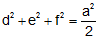Problem 259. Equilateral Triangle, Incircle, Tangency Points, Side, Distances, Squares The figure shows an equilateral triangle ABC. If a point D is on the incircle, E, F, and G are the points of tangency, DE = d, DG = e, DF = f, and AC = a, prove that. View or post a solution.See also:
 Home | Sitemap | Geometry | Problems | 251-260 | View or post a solution